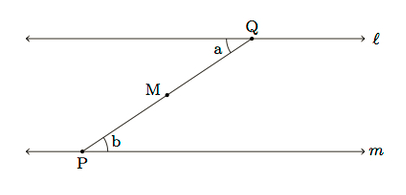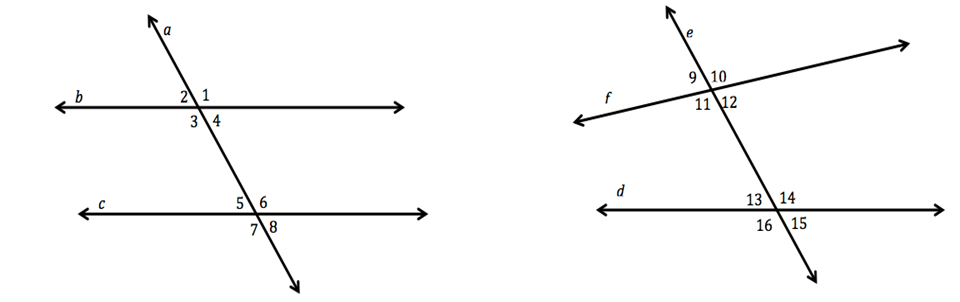Match Fishtank is now Fishtank Learning!

# Transformations and Angle Relationships

## Objective

Define and identify alternate interior and alternate exterior angles in parallel line diagrams. Find missing angles in parallel line diagrams.

## Common Core Standards

### Core Standards

?

• 8.G.A.2 — Understand that a two-dimensional figure is congruent to another if the second can be obtained from the first by a sequence of rotations, reflections, and translations; given two congruent figures, describe a sequence that exhibits the congruence between them.

• 8.G.A.5 — Use informal arguments to establish facts about the angle sum and exterior angle of triangles, about the angles created when parallel lines are cut by a transversal, and the angle-angle criterion for similarity of triangles. For example, arrange three copies of the same triangle so that the sum of the three angles appears to form a line, and give an argument in terms of transversals why this is so.

?

• 7.G.B.5

## Criteria for Success

?

1. Prove that alternate interior angles are congruent using a rotation.
2. Prove that alternate exterior angles are congruent using a translation and reflection.
3. Given an angle measure in a parallel line diagram, find other angle measurements.

## Tips for Teachers

?

• Lesson 18 builds on Lesson 17 by introducing two new angle relationships in parallel line diagrams: alternate interior and alternate exterior angles. Students use their knowledge of transformations to prove why these angles are congruent and to deepen their understanding of the relationships of angles in parallel line diagrams.
• The following materials may be useful for this lesson: patty (transparency) paper and protractors.

#### Remote Learning Guidance

If you need to adapt or shorten this lesson for remote learning, we suggest prioritizing Anchor Problems 1 and 2 (benefit from worked examples). Find more guidance on adapting our math curriculum for remote learning here.

#### Fishtank Plus

• Problem Set
• Student Handout Editor
• Vocabulary Package

## Anchor Problems

?

### Problem 1

Suppose $l$ and $m$ are parallel lines, with $Q$ a point on $l$ and $P$ a point on $m$, as pictured below. Also labeled in  the picture is point $M$, the midpoint of $\overline{PQ}$, and two angles $a$ and $b$.How can you use a rotation to prove that angles $a$ and $b$ are congruent?

#### References

Illustrative Mathematics Congruence of Alternate Interior Angles via Rotations

Congruence of Alternate Interior Angles via Rotations, accessed on Oct. 13, 2017, 4:15 p.m., is licensed by Illustrative Mathematics under either the CC BY 4.0 or CC BY-NC-SA 4.0. For further information, contact Illustrative Mathematics.

Modified by Fishtank Learning, Inc.

### Problem 2

In the picture below, $m$ and $n$ are parallel lines and angles $a$ and $b$ are shown. Show that angle $a$ is congruent to angle $b$ using rigid motions.#### References

Illustrative Mathematics Rigid Motions and Congruent Angles

Rigid Motions and Congruent Angles, accessed on Oct. 13, 2017, 4:16 p.m., is licensed by Illustrative Mathematics under either the CC BY 4.0 or CC BY-NC-SA 4.0. For further information, contact Illustrative Mathematics.

Modified by Fishtank Learning, Inc.

### Problem 3

In the diagrams below, lines $b$ and $c$ are parallel; lines $f$ and $d$ are not parallel.1. Identify all of the angles congruent to ${{\angle 1}}$ in the first diagram. Explain why each angle is congruent to ${{\angle 1}}$.
2. Identify all of the angles congruent to ${{\angle 1}}0$ in the second diagram. Explain why each angle is congruent to ${{\angle 1}}0$.

## Problem Set

?The following resources include problems and activities aligned to the objective of the lesson that can be used to create your own problem set.

• Examples where students are given one angle measure in a parallel line diagram and asked to determine all of the other angle measures with a brief explanation of how they determined the measurement

?

Use the diagram to answer the two questions that follow. In the diagram, lines ${{{L_1}}}$ and ${{{L_2}}}$ are intersected by transveral $m$, forming angles 1-8, as shown.1. If ${{{L_1}}}$ and ${{{L_2}}}$ are parallel, what do you know about ${\angle 2}$ and ${\angle 6}$? Use informal arguments to support your claim.
2. If ${{{L_1}}}$ and ${{{L_2}}}$ are parallel, what do you know about ${\angle 1}$ and ${\angle 3}$? Use informal arguments to support your claim.

#### References

EngageNY Mathematics Grade 8 Mathematics > Module 2 > Topic C > Lesson 12Exit Ticket

Grade 8 Mathematics > Module 2 > Topic C > Lesson 12 of the New York State Common Core Mathematics Curriculum from EngageNY and Great Minds. © 2015 Great Minds. Licensed by EngageNY of the New York State Education Department under the CC BY-NC-SA 3.0 US license. Accessed Dec. 2, 2016, 5:15 p.m..

Modified by Fishtank Learning, Inc.

?